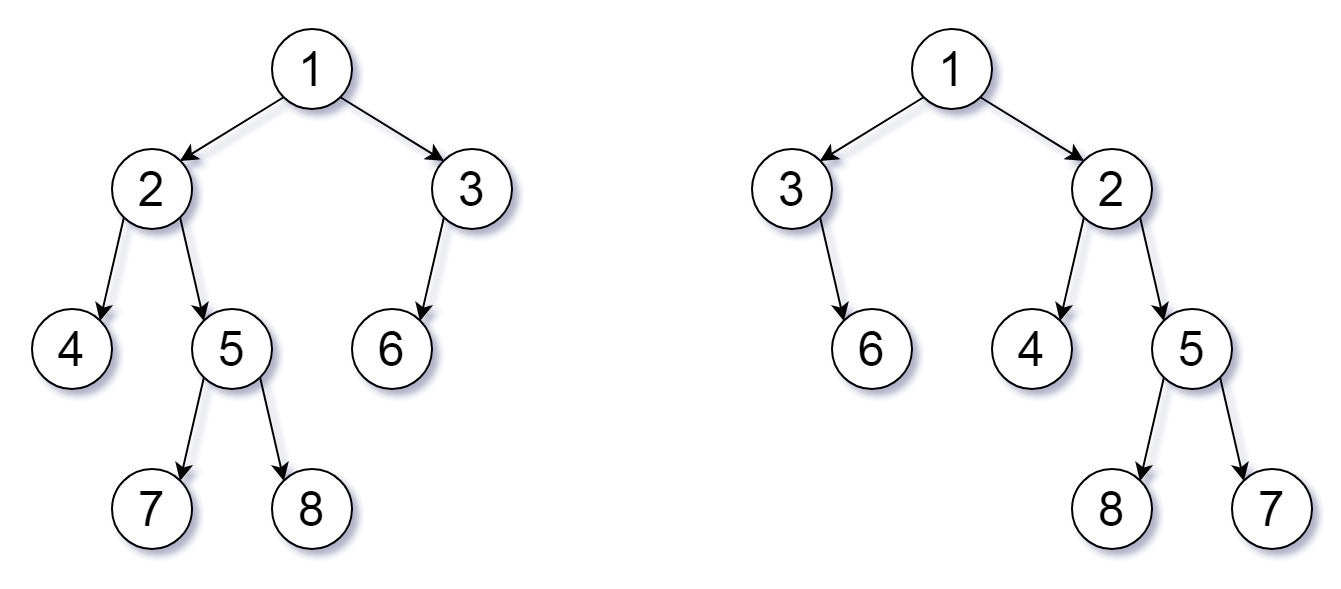# GeetCode Hub

For a binary tree T, we can define a flip operation as follows: choose any node, and swap the left and right child subtrees.

A binary tree X is flip equivalent to a binary tree Y if and only if we can make X equal to Y after some number of flip operations.

Given the roots of two binary trees `root1` and `root2`, return `true` if the two trees are flip equivelent or `false` otherwise.

Example 1:```Input: root1 = [1,2,3,4,5,6,null,null,null,7,8], root2 = [1,3,2,null,6,4,5,null,null,null,null,8,7]
Output: true
Explanation: We flipped at nodes with values 1, 3, and 5.
```

Example 2:

```Input: root1 = [], root2 = []
Output: true
```

Example 3:

```Input: root1 = [], root2 = 
Output: false
```

Example 4:

```Input: root1 = [0,null,1], root2 = []
Output: false
```

Example 5:

```Input: root1 = [0,null,1], root2 = [0,1]
Output: true
```

Constraints:

• The number of nodes in each tree is in the range `[0, 100]`.
• Each tree will have unique node values in the range `[0, 99]`.

/** * Definition for a binary tree node. * public class TreeNode { * int val; * TreeNode left; * TreeNode right; * TreeNode() {} * TreeNode(int val) { this.val = val; } * TreeNode(int val, TreeNode left, TreeNode right) { * this.val = val; * this.left = left; * this.right = right; * } * } */ class Solution { public boolean flipEquiv(TreeNode root1, TreeNode root2) { } }